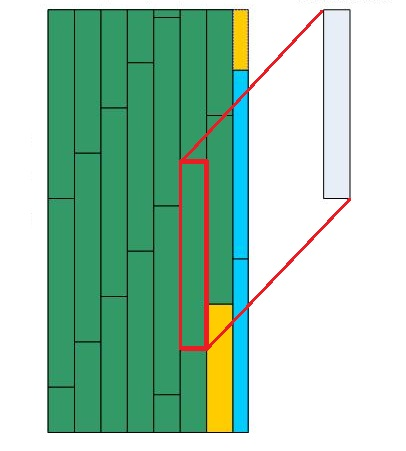# Laminate flooring. The calculation of the number of planks

The calculation of the number of planks requieredThis calculator should answer the laminate's question, "with the known size of one plank and area of a room, how many planks are needed, including the scraps."
Since I had already laid laminate twice :), the task was quite clear, and the calculator was created. A picture is worth a thousand words, so I've made one on the left. It actually depicts the method of laying on which the calculations are based.

Input data: the length and width of the room in meters, the length and width of the laminated plank in millimeters, the number of planks per pack, and how we lay the board (by length or by the width of the room)

Output data:
Room area - that's simple, just for reference
Number of planks used entirely - number of greens + yellow plank (in fact, it haы to be added the number of scraps or to the number of whole planks - I've decided to add them to the number of whole planks because the part shown in the picture below doesn't require lengthwise sawing.
Length of the last plank, millimeters - length of the yellow plank shown below
Number of scraps - number of blue planks
Scrap width, millimeters - width of the blue scraps
Total number of planks used - number of green planks + yellow plank + number of blue planks
Number of packages - as the laminate flooring is selling in packages - the necessary amount of packages
Number of unused planks - and that's the number of unused planks from the last package#### Laminate flooring. The calculation of the number of planks

Digits after the decimal point: 3
Room area, square meters

Number of planks used entirely

Length of the last plank, millimeters

Number of scraps

Scrap width, millimeters

Total number of planks used

Number of packages

Number of unused planks

URL copied to clipboard

#### Similar calculators

PLANETCALC, Laminate flooring. The calculation of the number of planks